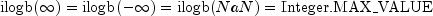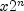JMSLTM Numerical Library 7.2.0
com.imsl.math

## Class IEEE

• ```public class IEEE
extends Object```
Pure Java implementation of the IEEE 754 functions as specified in IEEE Standard for Binary Floating-Point Arithmetic, ANSI/IEEE Standard 754-1985 (IEEE, New York).

This Java code is based on C code in the package fdlibm, which can be obtained from www.netlib.org. The original fdlibm C code contains the following notice.

Developed at SunSoft, a Sun Microsystems, Inc. business. Permission to use, copy, modify, and distribute this software is freely granted, provided that this notice is preserved.

• ### Method Summary

Methods
Modifier and Type Method and Description
`static double` ```copysign(double x, double y)```
Returns a value with the magnitude of x and with the sign bit of y.
`static boolean` `finite(double x)`
Finite number test on an argument of type double.
`static int` `ilogb(double x)`
Return the binary exponent of non-zero x.
`static boolean` `isNaN(double x)`
NaN test on an argument of type double.
`static double` ```nextAfter(double x, double y)```
Returns the next machine floating-point number next to x in the direction toward y.
`static double` ```scalbn(double x, int n)```
Returns 2n computed by exponent manipulation rather than by actually performing an exponentiation or a multiplication.
`static boolean` ```unordered(double x, double y)```
Unordered test on a pair of `double`s.
• ### Methods inherited from class java.lang.Object

`clone, equals, finalize, getClass, hashCode, notify, notifyAll, toString, wait, wait, wait`
• ### Method Detail

• #### copysign

```public static double copysign(double x,
double y)```
Returns a value with the magnitude of x and with the sign bit of y. If y is NaN then |x| is returned.
Parameters:
`x` - a `double` from which the magnitude will be gleaned
`y` - a `double` from which the sign will be gleaned
Returns:
a `double` value with magnitude x and sign of y
• #### finite

`public static boolean finite(double x)`
Finite number test on an argument of type double.
Parameters:
`x` - the `double` which is to be tested
Returns:
true if x is a finite number, false if x is a NaN or an infinity
• #### ilogb

`public static int ilogb(double x)`
Return the binary exponent of non-zero x.
Parameters:
`x` - a `double`
Returns:
an `int` representing the binary exponent of x. Special cases `ilogb(0) = -Integer.MAX_VALUE` and.
`Integer.MAX_VALUE`
• #### isNaN

`public static boolean isNaN(double x)`
NaN test on an argument of type double.
Parameters:
`x` - the `double` which is to be tested
Returns:
true if x is a NaN, false otherwise
• #### nextAfter

```public static double nextAfter(double x,
double y)```
Returns the next machine floating-point number next to x in the direction toward y.
Parameters:
`x` - a `double`
`y` - a `double`
Returns:
a `double` which represents the value which is closest to x in the interval bounded by x and y
• #### scalbn

```public static double scalbn(double x,
int n)```
Returns 2n computed by exponent manipulation rather than by actually performing an exponentiation or a multiplication.
Parameters:
`x` - a `double`
`n` - an `int` representing the power to which 2 is raised
Returns:
a `double` representing.
• #### unordered

```public static boolean unordered(double x,
double y)```
Unordered test on a pair of `double`s. Tests whether either of a pair of `double`s is a NaN.
Parameters:
`x` - a `double`
`y` - a `double`
Returns:
true if either x or y is a NaN, false otherwise
JMSLTM Numerical Library 7.2.0